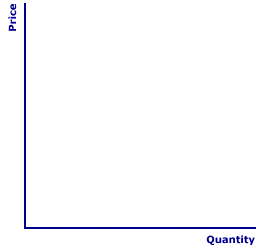Thursday  November 30, 2023
 AmosWEB means Economics with a Touch of Whimsy!MARKET ANALYSIS: The use of the market model to examine economic phenomenon involving demand, supply, prices, and exchanges. The simplest market analysis involves identifying equilibrium price and quantity, which is the point of intersection between the demand and supply curves. Some of the more useful market analysis, however, involves comparative static analysis of shifts in either the demand or supply curves, or both curves simultaneously. Other market analysis examines the consequences of price ceilings, price floors, and taxes.ELASTICITY AND SUPPLY INTERCEPT:

The intersection of a straight-line supply curve with vertical price axis and/or horizontal quantity axis reveals the relative price elasticity of supply. Intersection with the horizontal quantity axis means inelastic and intersection with the vertical price axis means elastic. Intersection with the origin means unit elastic supply.
The positioning of a supply curve relative to the price and quantity axes indicates the price elasticity of supply. Unlike demand, a straight-line supply curve does not contain all five elasticity alternatives. However, the elastic or inelastic nature of a supply curve is revealed by the intersection with the vertical price axis and/or horizontal quantity axis.

### Five Elasticity Alternatives

Five Supply CurvesThe elasticity of a supply curve depends on whether the supply curve intersects the price axis, the quantity axis, or the origin. The exhibit to the right can be used to illustrate the five elasticity alternatives.
• Perfectly Elastic: First up is perfectly elastic supply, which means an infinitesimally small change in price results in an infinitely large change in quantity supplied. The coefficient of elasticity for this alternative is E = ∞. A click of the [Perfectly E] button reveals a perfectly elastic supply curve, which is horizontal and intersects the vertical price axis.

• Relatively Elastic: Next up is relatively elastic supply, which means a relatively small change in price results in a relatively large change in quantity supplied. The coefficient of elasticity for this alternative is in the range of 1 < E < ∞. A click of the [Relatively E] button reveals a relatively elastic supply curve, which is flat but not horizontal and intersects the price axis.

• Unit Elastic: Third on the list is unit elastic supply, which means that any change in price is matched by an equal relative change in quantity. The coefficient of elasticity for this alternative is in the range of E = 1. A click of the [Unit Elastic] button reveals a unit elastic supply curve, which is a straight line extending from the origin.

• Relatively Inelastic: Next is relatively inelastic supply, which means a relatively large change in price is needed to induce a relatively small change in quantity supplied. The coefficient of elasticity for this alternative is in the range of 0 < E < 1. A click of the [Relatively In] button reveals a relatively inelastic supply curve, which is steep but not vertical and intersects the quantity axis.

• Perfectly Inelastic: Lastly is perfectly inelastic supply, means that quantity supplied is unaffected by any change in price. The coefficient of elasticity for this alternative is E = 0. A click of the [Perfectly In] button reveals a perfectly inelastic supply curve, which is vertical and intersects the horizontal quantity axis.

An explanation of this connection between elasticity and intercept can be had with a closer look at the elasticity concept. Elasticity is comparison between the percentage changes in price and quantity. A percentage change depends on both amount of the change, or the unit change, and the starting point, or base value, of the change. Because slope is constant for a straight-line supply curve, unit changes are always proportional for any of the supply curves displayed here.

The difference in elasticity rests with the base values. For a supply curve that intersects the vertical price axis, price starts with a larger base value than quantity. As such, any subsequent price change is relatively smaller, compared to this larger base, than the quantity change. This results in a relatively elastic supply.

For a supply curve that intersects the horizontal quantity axis, quantity starts with a larger base value than the price. As such, any subsequent quantity change is relatively smaller, compared to this larger base, than the price change. This results in a relatively inelastic supply.

For a supply curve that goes through the origin, price and quantity start with equal zero values, and equal bases. As such, all subsequent price and quantity changes are proportional. This results in a unit elastic supply.

 <= ELASTICITY AND DEMAND SLOPE ELASTICITY DETERMINANTS =>Recommended Citation:

ELASTICITY AND SUPPLY INTERCEPT, AmosWEB Encyclonomic WEB*pedia, http://www.AmosWEB.com, AmosWEB LLC, 2000-2023. [Accessed: November 30, 2023].

Check Out These Related Terms...

Or For A Little Background...

And For Further Study...
Search Again?GRAY SKITTERY[What's This?] Today, you are likely to spend a great deal of time going from convenience store to convenience store hoping to buy either a birthday greeting card for your grandmother or a coffee cup commemorating yesterday. Be on the lookout for empty parking spaces that appear to be near the entrance to a store.Your Complete ScopeAl Capone's business card said he was a used furniture dealer."Consult not your fears, but your hopes and your dreams. Think not about your frustrations, but about your unfulfilled potential. Concern yourself not with what you tried and failed in, but with what it is still possible for you to do. "-- Pope John XXIIIMPMarginal ProductA PEDestrian's Guide Xtra CreditTell us what you think about AmosWEB. Like what you see? Have suggestions for improvements? Let us know. Click the User Feedback link.| | | | | | | | | | |
| | | |

Thanks for visiting AmosWEB